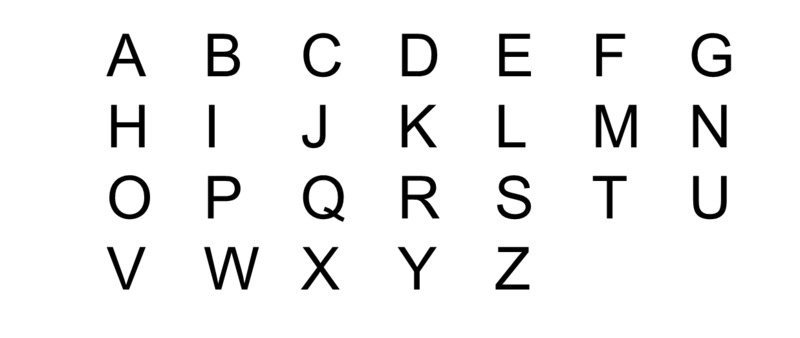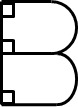# The Geometry of Letters

Alignments to Content Standards: 4.G.A.1

Letters can be thought of as geometric figures.1. How many line segments are needed to make the letter A? How many angles are there? Are they acute, obtuse, or right angles? Are any of the line segments perpendicular? Are any of the line segments parallel?

2. We can build all of these letters from line segments and arcs of circles. Build all of the capital letters with the smallest number of "pieces," where each piece is either a line segment or an arc of a circle.
3. Which letters have perpendicular line segments?
4. Which letters have parallel line segments?
5. Which letters have no line segments?
6. Do any letters contain both parallel and perpendicular lines?
7. What makes the lower case letters "i" and "j" different than all of the capital letters?

## IM Commentary

The purpose of this task is for students to analyze the geometry of letters. Letters provide a good opportunity for students to broaden their understanding of what constitutes a 2-dimensional geometric figure. Most students recognize polygons, circles, ellipses, and simple closed curves with some kind of symmetry as "shapes," but a 2-D geometric figure in general is simply a specified collection of points in the plane. So, for example, you can have a geometric figure that is not connected (like the lower case letters "i" and "j"), or you can have a geometric figure that is composed of a simple closed curve with a line segment sticking out of it (like the letter "P"). The benefit of working with letters as opposed to made-up figures with these characteristics is that students have already accepted them as objects, and so it seems reasonable to study them from a geometrical perspective.

This task has students composing and decomposing figures, which is an important way of looking at geometric figures and a skill that students start working on in kindergarten and continue building throughout elementary school and beyond. Students should have access to a plastic or metal tracing ruler with different sized circles, a straight edge, and some colored pencils or markers.

Students are often confused about whether two line segments are parallel or perpendicular. For example, parallel lines are lines that never meet. Does this mean that these line segments are parallel?Even though these segments do not meet, the answer is "no." The trick is to realize that every line segment is contained in an (infinite) line. So two line segments are parallel if and only if the lines that contain them are parallel. Likewise, two line segments are perpendicular if and only if the lines that contain them are perpendicular. This is what allows us to see that the letter F, for example, is composed of three line segments, two of which are parallel to each other and both of which are perpendicular to the third.

Note that whenever two lines segments meet at an endpoint, two angles are implicitly defined. Often students only focus on the angle that is less than 180 degrees, but for example, the letter L can be thought of as defining both an angle that is 90 degrees and one that is 270 degrees. It would be good to address this directly in a whole-class discussion.

## Solution

1. The letter A is composed of 3 line segments which meet in three places and form 5 angles less than 180 degrees. Three of these angles are acute, and two are obtuse. Note that students might also count angles that are greater than 180 degrees, so it is important for students to explicitly identify the angles they see. For example, a student might see an angle greater than 180 degrees at the top of the letter A.

Alternatively, the a student might see the letter A as being composed of 5 line segments and see two straight-angles on the sides of the letter A where the horizontal segment meets them. Of course, this isn't the smallest number of pieces needed to build the letter A, but it is a correct decomposition and analysis of it.

None of the line segments are perpendicular or parallel.

2. The hardest ones are the ones composed of both segments and curves. B can be made from 4 segments and two semi-circles, for example:3. These letters have perpendicular line segments:

B, D, E, F, H, L, P, R, T

For example, Here is an analysis of the letter B which is composed of 4 line segments (three of which are parallel to each other and are all perpendicular to the fourth) and two arcs of a circle:4. These letters have parallel line segments:

B, D, E, F, H, M, N, R, U, Z

5. These letters have no line segments: C, O, S. Depending on the font (or your handwriting), Q might or might not contain a line segment.
6. These letters have both parallel and perpendicular line segments:

B, D, E, F, H, R

7. The lower case letters "i" and "j" are different than all of the capital letters because they are disconnected. That is, they are made out of pieces that don't touch.